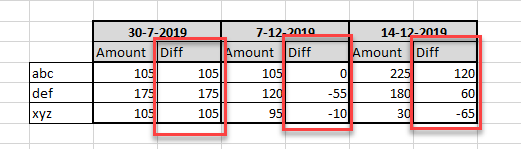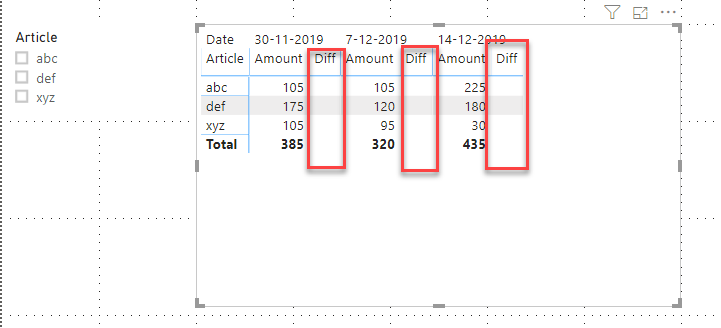cancel
Showing results for
Did you mean:Anonymous
Not applicable

## Calculate difference between values in different columns

Hi,

I would like to build the Excel visualization (see screenshot) in PBI. I assume this is possible with a DAX measure? I've been playing around with some DAX functions and tried some solutions from previous posts, but I cannot get my head around it.

It basically needs to calculate the difference in Amount compared to the Amount of the previous date. It should be dynamic; new dates can get added to the table.

Any hints, tips are appreciated.Best regards,

Armand

1 ACCEPTED SOLUTIONAnonymous
Not applicable

@az38 thank you for your proposal, but unfortunately it did not work out.

Eventually I found the solution using the following measures:

1. PreviousDate
2. Amount previous date
3. Diff with prev date
4. Diff %
``````PreviousDate =
VAR CurrentDate =
SELECTEDVALUE ( 'Sheet1'[Date] )
RETURN
CALCULATE (
MAX ( 'Sheet1'[Date] );
ALLSELECTED ( Sheet1 );
KEEPFILTERS ( Sheet1[Date] < CurrentDate )
)``````
``````Amount previous date =
VAR Prev = [PreviousDate]
RETURN
CALCULATE (
[Total Amount];
Sheet1[Date] = Prev
)``````
``````Diff with previous =
[Total Amount] - [Amount previous date]``````
``````Diff % =
IF (
ISBLANK ( [Diff with previous] );
BLANK ();
DIVIDE ( [Diff with previous]; [Amount previous date] ) * 100
)``````
2 REPLIES 2Community Champion

Hi @Anonymous

try a measure

``diff = SELECTEDVALUE('Table'[Amount])-calculate(FIRSTNONBLANK('Table'[Amount];1);filter(ALLEXCEPT('Table';'Table'[Article]);'Table'[Date]<selectedvalue('Table'[Date])))``

do not hesitate to give a kudo to useful posts and mark solutions as solution

do not hesitate to give a kudo to useful posts and mark solutions as solutionAnonymous
Not applicable

@az38 thank you for your proposal, but unfortunately it did not work out.

Eventually I found the solution using the following measures:

1. PreviousDate
2. Amount previous date
3. Diff with prev date
4. Diff %
``````PreviousDate =
VAR CurrentDate =
SELECTEDVALUE ( 'Sheet1'[Date] )
RETURN
CALCULATE (
MAX ( 'Sheet1'[Date] );
ALLSELECTED ( Sheet1 );
KEEPFILTERS ( Sheet1[Date] < CurrentDate )
)``````
``````Amount previous date =
VAR Prev = [PreviousDate]
RETURN
CALCULATE (
[Total Amount];
Sheet1[Date] = Prev
)``````
``````Diff with previous =
[Total Amount] - [Amount previous date]``````
``````Diff % =
IF (
ISBLANK ( [Diff with previous] );
BLANK ();
DIVIDE ( [Diff with previous]; [Amount previous date] ) * 100
)``````